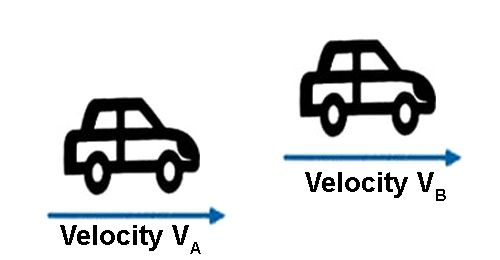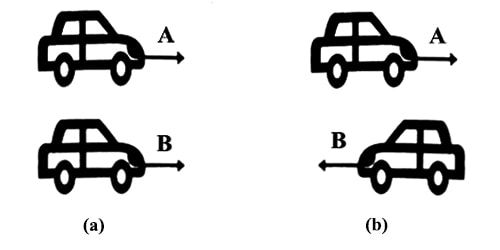# Motion in the Same Direction

Motion in the same direction

When two bodies move in the same direction along a straight line, then the relative velocity of A with respect to B will be equal to the difference between the two velocities.

Example: Look at the two figures below. In the first figure two cars are moving in the same direction [Fig (a)] and in the second figure [Fig (b)] they are moving opposite to each other. How will you determine the relative velocity between them? Here, in the case of two parallel linear motion, the following two motions are to be considered for determining relative velocity.That is, vAB = vA – vB, if vA > vB, then the direction of vAB will be along the direction of vA. So looking from B the body ‘A’ will appear moving in the forward direction with velocity vAB. Again, if it is seen from A the body B will appear to be moving backward with velocity vBA (= – vAB) as the direction of vBA is opposite to vAB.

Conclusions

Following conclusions may be drawn from the above example:

(1) If two bodies move in the same direction, then by subtracting their velocities relative velocity can be obtained.

(2) If two bodies move in the opposite direction, then the relative velocity is obtained by addition of the velocities.